Each question requests a specific type of answer. Usually a question will display a hint at the end of the question as to what type of answer is expected. In addition to multiple choice questions and other standard types, this system also features several mathematical answer types. Read on for suggestions on entering answers for these types.

Some question ask for numerical answers. Acceptable answers include whole numbers, integers (negative numbers), and decimal values.

In special cases, you may need to enter DNE for "Does not exist", oo for infinity, or -oo for negative infinity.

If your answer is not an exact value, you'll want to enter at least 3 decimal places unless the problem specifies otherwise.

### Fractions and Mixed Numbers

Enter fractions like 2/3 for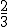. If not specified, the fraction does not need to be reduced, so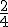is considered the same as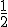.

For a reduced fraction answer, be sure to reduce your fraction to lowest terms. Ifis the correct answer,is not reduced, and would be marked wrong.

For both fraction and reduced fraction type problems, improper fractions are OK, like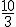, but mixed numbers will not be accepted.

For a mixed number problem, enter your answer like 5_1/3 for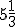. Improper fractions will not be accepted. Also, be sure to reduce the fractional portion of the mixed number to lowest terms.

### Calculations

Some questions allow calculations be entered as answers. You can also enter whole numbers, negative numbers, or decimal numbers. If you enter a decimal value, be sure to give at least 3 decimal places.

Alternatively, you can enter mathematical expressions. Some examples:

EnterTo get
sqrt(4)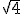= 2
2/(5-3)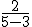= 1
3^2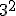= 9
sin(pi)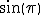= 0
arctan(1)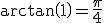(note: tan^-1(1) will not work)
log(100)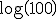= 2

Note that when entering functions like sqrt and sin, use parentheses around the input. sin(3) is ok, but sin3 is not.

You can use the Preview button to see how the computer is interpreting what you have entered

### Algebraic Expressions

Some questions ask for algebraic expression answers. With these types of questions, be sure to use the variables indicated. In your answer, you can also use mathematical expressions for numerical calculations.

Examples:

TypeTo get
-3x^2+5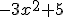(2+x)/(3-x)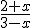sqrt(x-5)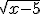3^(x+7)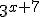1/(x(x+1))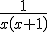5/3x+2/3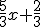sin(pi/3x)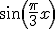ln(x)/ln(7)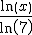arcsin(x)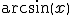With any function like sqrt, log, or sin, be sure to put parentheses around the input: sqrt(x+3) is OK, but sqrtx+3 or sqrt x+3 is not.

Note that the shorthand notation sin^2(x) will display correctly but not evaluate correctly; use (sin(x))^2 instead.

Unless the problem gives specific directions, any algebraically equivalent expression is acceptable. For example, if the answer was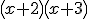, then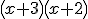and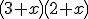would also be acceptable.

You can use the Preview button to see how the computer is interpreting your answer. If you see "syntax ok", it means the computer can understand what you typed (though it may not be the correct answer). If you see "syntax error", you may be missing a parenthese or have used the wrong variables in your answer.